The OpenFOAM FoundationzeroField Class Reference

A class representing the concept of a field of 0 used to avoid unnecessary manipulations for objects which are known to be zero at compile-time. More...

Inheritance diagram for zeroField: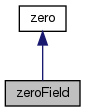[legend]
Collaboration diagram for zeroField:[legend]

## Public Member Functions

zeroField ()
Construct null. More...

scalar operator[] (const label) const

zeroField field () const

zeroField operator() () const

zeroField operator- () constPublic Member Functions inherited from zero
zero ()
Construct null. More...

operator bool () const
Return 0 for bool. More...

operator label () const
Return 0 for label. More...

operator float () const
Return 0 for float. More...

operator double () const
Return 0 for double. More...

operator long double () const
Return 0 for double. More...Public Types inherited from zero
typedef zero value_type

## Detailed Description

A class representing the concept of a field of 0 used to avoid unnecessary manipulations for objects which are known to be zero at compile-time.

Used for example as the argument to a function in which certain terms are optional, see source terms in the MULES solvers.

Definition at line 50 of file zeroField.H.

## ◆ zeroField()

 zeroField ( )
inline

Construct null.

Definition at line 59 of file zeroField.H.

Here is the call graph for this function: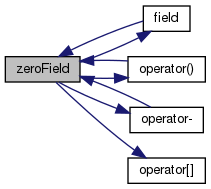Here is the caller graph for this function: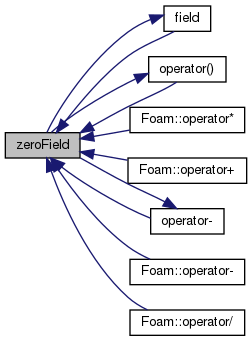## ◆ operator[]()

 Foam::scalar operator[] ( const label ) const
inline

Definition at line 30 of file zeroFieldI.H.

Referenced by zeroField::zeroField().

Here is the caller graph for this function: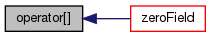## ◆ field()

 Foam::zeroField field ( ) const
inline

Definition at line 36 of file zeroFieldI.H.

References zeroField::zeroField().

Referenced by zeroField::zeroField().

Here is the call graph for this function: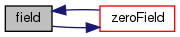Here is the caller graph for this function: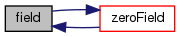## ◆ operator()()

 Foam::zeroField operator() ( ) const
inline

Definition at line 42 of file zeroFieldI.H.

References zeroField::zeroField().

Referenced by zeroField::zeroField().

Here is the call graph for this function: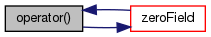Here is the caller graph for this function: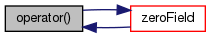## ◆ operator-()

 Foam::zeroField operator- ( ) const
inline

Definition at line 48 of file zeroFieldI.H.

References zeroField::zeroField().

Referenced by zeroField::zeroField().

Here is the call graph for this function: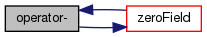Here is the caller graph for this function: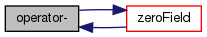The documentation for this class was generated from the following files: